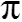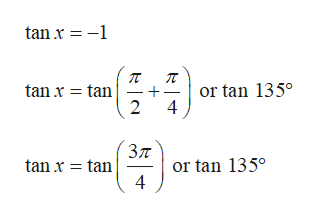# Hello, I need help understanding how to form a general formula, using f(x)=5 tan x, for all the solutions to f(x)=-5 by using angle(s) in the interval [0,pi) and adding multiples of some integer k. I do not know how to input -5 into 5 tan x, or how to begin.

Question
45 views

Hello,

I need help understanding how to form a general formula, using f(x)=5 tan x, for all the solutions to f(x)=-5 by using angle(s) in the interval [0,pi) and adding multiples of some integer k. I do not know how to input -5 into 5 tan x, or how to begin.

check_circle

star
star
star
star
star
1 Rating
Step 1

Consider the provided function.

Step 2

The above information can be written as:

Step 3

The provided interval is [0,). The value of tan 1350 = -1 because tan 1350 is lying on 2nd quadrant where tan x is negative.

...help_outlineImage Transcriptionclosetan x 1 tan x tan or tan 135° 2 4 tan x tan or tan 135° 4 fullscreen

### Want to see the full answer?

See Solution

#### Want to see this answer and more?

Solutions are written by subject experts who are available 24/7. Questions are typically answered within 1 hour.*

See Solution
*Response times may vary by subject and question.
Tagged in

### Other